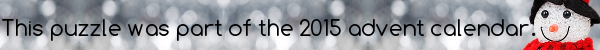mscroggs.co.uk
mscroggs.co.uksubscribe

## 13 DecemberPut the digits 1 to 9 (using each digit once) in the boxes so that the three digit numbers formed (reading left to right and top to bottom) have the desired properties written by their rows and columns.
The row marked sum is equal to the sum of the other two rows. The column marked sum is equal to the sum of the other two columns.
 odd all digits even sum even odd sum
Today's number is the largest three digit number in this grid.
Tags: numbers, grids

## Archive

Show me a random puzzle
▼ show ▼